Students can Download Maths Chapter 1 Number System Additional Questions and Answers, Notes Pdf, Samacheer Kalvi 7th Maths Book Solutions Guide Pdf helps you to revise the complete Tamilnadu State Board New Syllabus and score more marks in your examinations.

## Tamilnadu Samacheer Kalvi 7th Maths Solutions Term 3 Chapter 1 Number System Additional Questions

Additional Questions and Answers

Exercise 1.1

Question 1.
Match the following: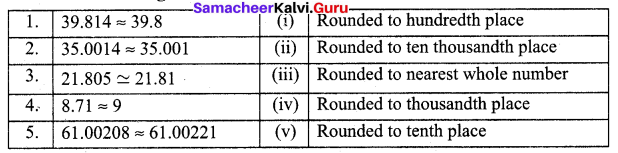Solution:
1 – (v)
2 – (iv)
3 – (i)
4 – (iii)
5 – (ii)

Question 2.
Round 89.357 to the nearest whole number.
Solution:
Underlining the digit to be rounded 89.357. Since the digit next to the underlined digit 3 which is less than 5, the underlined digit remains the same.
∴ The nearest whole number 89.357 rounds to 89.

Question 3.
Round 110.929 to the nearest tenths place.
Solution:
Underlining the digit to be rounded 110.929. Since the digit next to the underlined digit is 2 which is less than 5.
∴ The underlined digit 9 remains the same. Hence the rounded number is 110.9

Question 4.
Round 87.777 upto 2 places of decimal.
Solution:
Rounding 87.777 upto 2 places of decimal means round to the nearest hundredths place. Underlining the digit in the hundredth place of 87.777 gives 87.777. Since the digit after the hundredth place value is 7 which is more than 5, we add 1 to the underlined digit. So the rounded value of 87.777 upto 2 places of decimal is 87.78Exercise 1.2

Question 1.
If Sheela bought 2.083 kg of grapes and 3.752 kg of orange. What is the total weight of fruits
Solution: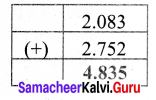Weight of grapes = 2.083 Kg
Weight of orange = 2.752 Kg
Total weight = (2.083 + 2.752) Kg = 4.835 Kg

Question 2.
Kathir bought 8.72 kg of sugar, 7.302 kg of grains. His carry bag can contain only 15 kg of weight. What is the remaining weight of items bought?
Solution: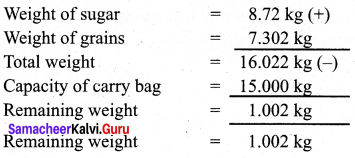Question 3.
Use place value grid to add 7.357 and 13.92.
Solution:
Let as use place value grid.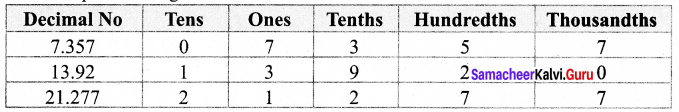Exercise 1.3

Question 1.
Cost of 1m cloth is ₹ 6.75. Find the cost of 14.75m correct to two places of decimal.
Solution: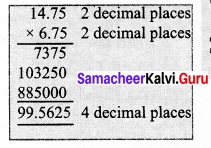Cost of 1 m cloth = ₹ 6.75
Cost of 14.75 m cloth = 14.75 × 6.75
= ₹ 99.5625
= ₹ 99.56Question 2.
Length of a side of a square is 18.35 cm. Find its Area.
Solution: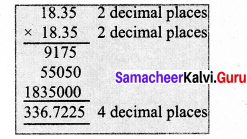Side of a square = 18.35 cm
Area of a square = (Side × Side) sq.units
= 18.35 × 18.35 cm2
= 336.7225 cm2

Exercise 1.4

Question 1.
A wire of length 363.987m is cut into 30 pieces. What is the length of each piece?
Solution:
Length of the wire = 363.987m
i.e Total length of 30 pieces = $$\frac { 363987 }{ 1000 }$$ m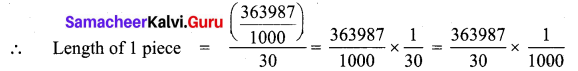∴ Length of 1 piece
= 12132.9 × $$\frac { 1 }{ 1000 }$$
Length of 1 piece of wire = 12.1329 m

Question 2.
A cake of 50kg needs 23.4 kg sugar. Find the weight of cake made by 1 kg of sugar.
Solution:
Weight of cake made using 23.4 kg sugar = 50 kg
Weight of cake made using 1 kg sugar = $$\frac { 50 }{ 23.4 }$$
= $$\frac { 50 }{ 23.4 }$$ x $$\frac { 10 }{ 10 }$$ = $$\frac { 500 }{ 234 }$$ = 2.1367 Kg
= 2.14 Kg
Weight of cake made using 1 kg sugar = 2.14 KgQuestion 3.
A pack of 20 pencils cost ₹ 94.4. What is the cost of each pencil?
Solution:
Cost of 20 pencils = ₹ 94.4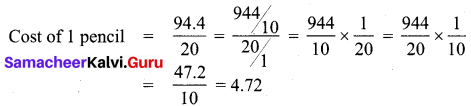∴ Cost of 1 pencil = ₹ 4.72